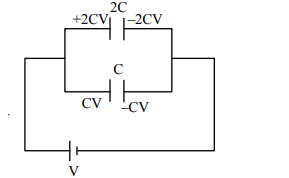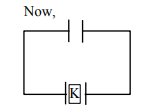# Two capacitors of capacities 2C and C are joined in parallel and charged up to potential V.Question:

Two capacitors of capacities 2C and C are joined in parallel and charged up to potential V. The battery is removed and the capacitor of capacity C is filled completely with a medium of dielectric constant K. The potential difference across the capacitors will now be :

1. $\frac{\mathrm{V}}{\mathrm{K}+2}$

2. $\frac{\mathrm{V}}{\mathrm{K}}$

3. $\frac{3 \mathrm{~V}}{\mathrm{~K}+2}$

4. $\frac{3 \mathrm{~V}}{\mathrm{~K}}$

Correct Option: , 3

Solution:$V_{C}=\frac{2 C V+C V}{K C+2 C}$

$=\frac{3 V}{K+2}$